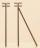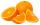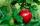# Fractions + expression of a variable from the formula - math problems

#### Number of problems found: 56

• Simple equation 324 = n • 27, solve for n
• Two thirdsFind two-thirds of the number equal to two-thirds of 99
• Find unknown 2Find unknown denominator: 2/3 -5/? = 1/4
• Interior anglesCalculate the interior angles of a triangle that are in the ratio 2: 3: 4.How many times does the surface of a sphere decrease if we reduce its radius twice?
• Above EarthTo what height must a boy be raised above the earth to see one-fifth of its surface.
• A fractionI think of a fraction, if we increase its numerator and denominator by one, the value of the fraction increases by one tenth, what fraction do I think?
• CoinsThe money - coins are minted from the hardest bronze, which contains copper and tin in a ratio of 41: 9. How much copper and tin are in 2kg of bronze money?
• The poleThe telegraph pole is supported by a 4 m bullet at 3/4 of its height, the end of which is at a distance 2.5 m from the pole post. Calculate the height of the telegraph pole.
• FamilySon is 3/5 smaller than the mother. Mother is 99 cm higher than the son. How tall is the mother?
• Hectoliters of waterThere are 942 hectoliters of water in a cylindrical tank with an inner diameter of 6 m. The water reaches two thirds of the depth of the tank. Calculate its depth.
• Marriage sttusIn our city, there are 3/5 of the women married to 2/3 of the men. Find what part of the population is free.
• LibraryNew books were purchased for the library. Five-eighths were professional books, one-fifth were encyclopedias, and 231 books were dictionaries. How many professional books were there?
• Right triangle from axesA line segment has its ends on the coordinate axes and forms with them a triangle of area equal to 36 square units. The segment passes through the point ( 5,2). What is the slope of the line segment?
• The angles ratioThe angles in the ABC triangle are in the ratio 1: 2: 3. find the sizes of the angles and determine what kind of a triangle it is.
• SSA and geometryThe distance between the points P and Q was 356 m measured in the terrain. The PQ line can be seen from the viewer at a viewing angle of 107° 22 '. The observer's distance from P is 271 m. Determine the viewing angle of P and observer.
• OrangesMother divided her three children's oranges in a ratio of 6:5:4. Two children gave 45 oranges. How many oranges were there?
• Water containerThe cube-shaped container is filled to two-thirds of its height. If we pour 18 liters, it will be filled to three-fifths of the height. What is the volume of the whole container?
• Two thirdsTwo thirds of the unknown number is 8/7. What is 7/3 of this number?
• GardenFather dig up the garden in 9 hours. Son in 13 hours. How many hours take dig up the garden together?

Do you have an interesting mathematical word problem that you can't solve it? Submit a math problem, and we can try to solve it.

We will send a solution to your e-mail address. Solved examples are also published here. Please enter the e-mail correctly and check whether you don't have a full mailbox.

Please do not submit problems from current active competitions such as Mathematical Olympiad, correspondence seminars etc...

Need help to calculate sum, simplify or multiply fractions? Try our fraction calculator. Fraction Word Problems. Expression of a variable from the formula - math problems.